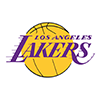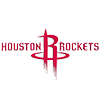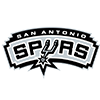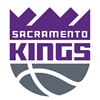DraftKings Western Conference Odds
+125
1.25 to 1Lakers
37.6% implied probability

+275
2.75 to 1Clippers
22.6% implied probability

No changes have been recorded yet.
+900
9 to 1Nuggets
8.5% implied probability

+1000
10 to 1Mavericks
7.7% implied probability

+1200
12 to 1Jazz
6.5% implied probability

+2000
20 to 1Suns
4.0% implied probability

+2000
20 to 1Trail Blazers
4.0% implied probability

+3300
33 to 1Warriors
2.5% implied probability

+5000
50 to 1Rockets
1.7% implied probability

+5000
50 to 1Pelicans
1.7% implied probability

+8000
80 to 1Grizzlies
1.0% implied probability

No changes have been recorded yet.
+8000
80 to 1Spurs
1.0% implied probability

No changes have been recorded yet.
+15000
150 to 1Kings
0.6% implied probability

+25000
250 to 1Timberwolves
0.3% implied probability

+25000
250 to 1Thunder
0.3% implied probability